Reach Us+441474556909
Linearization of Power Amplifier using the Modified Feed Forward Method | OMICS International
Journal of Textile Science & Engineering
All submissions of the EM system will be redirected to Online Manuscript Submission System. Authors are requested to submit articles directly to Online Manuscript Submission System of respective journal.

# Linearization of Power Amplifier using the Modified Feed Forward Method

Motavalli MR1* and Solbach K2

1Department of Communication Technique, Faculty of Engineering, University of Qom, Qom, Iran

2Department of Microwave and RF-Technology, Faculty of Engineering, University of Duisburg-Essen, Duisburg, Germany

*Corresponding Author:
Motavalli MR
Department of Communication Technique
Faculty of Engineering, University of Qom
Qom, Iran
Tel: +98 25 3210 3732
E-mail: [email protected]

Received date: January 24, 2017; Accepted date: February 20, 2017; Published date: February 27, 2017

Citation: Motavalli MR, Solbach K (2017) Linearization of Power Amplifier using the Modified Feed Forward Method. J Textile Sci Eng 6:286. doi: 10.4172/2165- 8064.1000286

Copyright: © 2017 Motavalli MR, et al. This is an open-access article distributed under the terms of the Creative Commons Attribution License, which permits unrestricted use, distribution, and reproduction in any medium, provided the original author and source are credited.

Visit for more related articles at Journal of Textile Science & Engineering

#### Abstract

A modified circuit for improving linearization of power amplifier based on the model of the Feed Forward circuit amplifier is proposed. With the help of mathematical model for the single power amplifier, the circuit is simulated and a demonstrator is fabricated and measured complex Taylor series are used for modeling the power amplifier by the approximation of the amplitude transfer function and the level-dependence of the transmission-phase of the power amplifier. This can be understood as a simplified form of volterra series. In our proof of concept experiment, we verified the concept but also found that the adjustment of the circuit is critically dependent on the drive conditions and linearization is achieved only for a narrow range of drive power. The proposed circuit in compared with the conventional Feed Forward amplifier in addition a significant increase in efficiency, to minimize the power of the distortion signal 3IMD-products at high drive levels.

#### Keywords

Volterra series; Complex taylor series; 3IMD-products, pre-distortion, Feed Forward amplifier; Power combiner

#### Introduction

The use of high power amplifiers with high linearity for mobile and satellite communications systems is essential. For example, in second and third generation mobile systems, GSM (Global System Mobile), 3GPP (third Generation Partnership Project), a large number of signals with different frequencies are transmitted from BS (Base Station) by high power amplifiers at the same time . To avoid interference of these amplified signals, the amplifier must operate linear and that linearization is not possible by classical methods. Since the amplitude of intermodulation signals at the output of the amplifiers depends on the size of the input signals, input signals with large amplitude limit the performance of the amplifier. If the connection between an amplifier input and output signals display as transformation function that is extended in the form of a series (for example Taylor’s series), we see that for the larger signal, the role of higher degrees of expression is more and more important, that is, the behavior of amplifiers is no longer linear and amplifier operates in saturation (non-linear) region. The saturation region, due to high output power and resulting high efficiency in mobile communications and satellite systems play an important role. Intermodulation signals with large amplitudes produce in this region of amplifier which leads to large distortion in output. Generally, nonlinearity in an amplifier can appear in two different forms: first production of new frequency components in the output of the amplifier and second dependence of gain amplitude and phase of the amplifiers to amplitude of input signals. If amplifiers have been multiple input signals frequency a type of distortion signals, that is, 3IMD-products (third order Intermodulation Distortion) should be considered more than other produced signals in output of amplifier, because they are near to frequency of original signals (input signals). They are in the range of useful bandwidth amplifiers and due to limitations in fabrication are not removable in practice .

The distortion signal of type 3IMD-products in base stations are propagated by high power amplifier in total send bandwidth and cause distortion and interference in band of inside channel as well as the neighboring channels. This problem occurs even on TV channels (by 3IMD-products and even 2IMD-products), where a large number of channels have placed at a close frequency near each other. The aim of this paper is to design a concept for a power amplifier with high linearity and high efficiency.

This paper presented the proposed circuit for improving linearization of the power amplifier based on the model of the Feed Forward circuit amplifier. In section 2, the principle of operation of the amplifier concept is discussed. A mathematical model of new amplifier concept is proposed in section 3. In section 4, a simulation model is used to investigate in detail the signals within the circuit and the performance and limitations of the amplifier. Finally in section 5, experimental proof of new amplifier concept is presented. Simulation and measurement results are compared and show good agreement.

#### New Amplifier Design

The classical parallel power combiner amplifier using two equal linear amplifiers have been used for many years in order to efficiently produce higher output power levels (doubles the available output power of one single amplifier) and also in order to improve the reliability and availability of the amplifier system component. However, linearity of amplification of each individual amplifier is not improved over the individual amplifiers. Power-added efficiency of the combiner amplifier circuit can be high when the amplifiers are driven close to the saturation level and consequently at high intermodulation level. Another successful concept in linearization of power amplifiers is pre-distortion, which can yield higher power efficiency, yet at lower cancellation ratios of unwanted products [3-6]. On the other hand, amplifiers for very high linearity requirements in mobile communications successfully employ the feed forward (FF) – amplifier scheme, Figure 1, which cancels the nonlinear intermodulation-products (IM) of a high power primary amplifier by superposition of signals from an auxiliary amplifier [7- 11]. However, this concept suffers from a degradation of the efficiency of the amplifier which is mainly due to the linearity requirements on the auxiliary amplifier. The FF-amplifier entails a first loop to extract the intermodulation distortion components from the power amplifier output while the second loop inverts phase and amplifies this signal in an auxiliary amplifier such that it destructively superimposes and cancels the original intermodulation signal at the output coupler. Distortions from the auxiliary amplifier have to be kept very low so that this amplifier needs to be operated far off saturation which means high dc power requirement. Since the auxiliary amplifier cannot contribute to the fundamental signal output power, its supply power degrades the power-added efficiency of the FF-amplifier [12-13] and also since the auxiliary amplifier is driven at low input power (low amplitude), a good ratio of cancelling of the 3IMD-products signals for small amplitude obtained, in other words, cancelling of the 3IMD-products signals for high output power is extremely low.

While the FF-amplifier has found wide application in communication systems due to its superior intermodulation suppression, its efficiency problem has inspired a modified concept which allows the auxiliary amplifier to contribute fundamental signal power in addition to cancelling the intermodulation products. The new circuit, Figure 2, exhibits a two-loop topology of a conventional feed forward amplifier, however, both loops are modified and the two amplifiers are assumed to be identical power amplifiers. In the first loop, the input power divider splits the input signal in a 1:3 ratio while at the output, the combiner is 1:1. The first loop acts as a pre-distortion stage while the second is a distortion cancelling and power combining loop.

For the presentation of the circuit’s operation principle, we assume that the fundamental input voltage signal of the circuit is sin (t). When the first power splitter divides this signal at a ratio of 1 to 3, the input signal to the upper power amplifier A is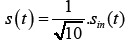while the signal incident to the lower power amplifier B is 3. sin (t). The two amplifiers are assumed to be identical with equal amplification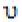and distortion products s′ (t) and s′′(t) for power amplifier A and B, respectively, under identical drive conditions.

The output voltage of power amplifier A is a superposition of the fundamental signal amplified by voltage gain v and a distortion product: v.s(t)+s′(t). The upper coupler samples this combined signal and feeds it to the lower coupler in front of amplifier B. Assuming both coupling coefficients as jk (note: complex notation used to indicate a phase shift of 90°), the sampled signal offered to the input of amplifier B is k2.v.s(t)-k2.s′(t) which is combined with the input signal 3.s(t) after its travel through the lower delay line to give the total voltage signal, (3-k2.v).s(t)-k2.s′(t) Note, the coupler and delay line insertion loss have been neglected for simplification and time delay of the both power amplifiers is compensated by two delay line in two-loop).

With our aim to drive the lower amplifier at the same fundamental signal level as the upper amplifier, we choose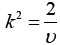to achieve the total voltage signal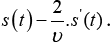It is seen that this lower amplifier input signal is a pre-distorted version of the input signal of the upper amplifier, with the distortion component as a replica of the upper amplifier distortion, but reversed in phase. Assuming the distortion component to be much smaller than the fundamental component, the lower amplifier will amplify the fundamental signal plus its distortion component by the voltage amplification υ. In addition, in the same way as the upper amplifier, the lower amplifier will produce a distortion component due to its fundamental signal excitation. The resulting output signal of amplifier B is then v.s(t)-2.s′(t)+s′(t). Assuming perfectly equal amplifiers A and B, the distortion signal originating from the lower amplifier is equal to the one generated by the upper amplifier, such that the output signal of the amplifier B is v.s(t)-s′(t).

In the upper signal path, the output signal of the power amplifier A travels through an upper delay line by neglecting the delay line’s attenuation, appears at the combiner as: v.s(t)+s′(t). By comparing signals in the upper and lower path, we see that the fundamental signals are equal and thus can be combined to give double power while the distortion components incident to the combiner are equal and in antiphase and thus cancel to give the total output power: v.s(t)+s′(t).

It has to be noted that this analysis is over-simplified with the assumption of perfect identity of the two amplifiers and it neglects the effect of the increased driving level of the lower amplifier due to the added pre-distortion signal. With slightly unequal amplifiers and slightly unequal driving levels, it is still possible to achieve nearperfect cancellation of intermodulation at the price of a loss in the power combining efficiency, as can be shown by simulation, section 4. However, a major performance limitation of the new circuit was found by analysis of an experimental amplifier system in section 5, to originate in the drive power level dependence of the amplifier voltage gain (magnitude and phase) and the insertion loss of the delay lines.

#### Mathematical Model

Characterization of single power amplifiers

To describe the behavior of the entire circuit mathematically, each power amplifier should be first characterized separately, it means that each power amplifier should be represented as a mathematical model; all other components can be described by simple mathematical models, since their behavior can be assumed to be linear in the region of interest.

For nonlinear behavior of power amplifier, the power transfer function has been measured, in the other words, the behavior of each amplifier is measured regarding 1 dB compression point and intermodulation products of orders 3, 5, 7 and 9 (3IMD-products, 5IMD-products, 7IMD-products and 9IMD-products) separately. To develop the mathematical description of this behavior, Amplifier output voltage to the input voltage is expanded in a series. At first, Taylor series is used, that due to stark non-linearity, model obtained was not fit to actual behavior of amplifiers. Next the volterra series is used that relation other series is more flexible and for description nonlinearity systems is more appropriate [14-16]. The measurements of the characteristics of the power transfer function has been done with two tone input signal. In the calculations performed, it has been demonstrated that if both amplitudes input signals are equal (such as common case in GSM), volterra series becomes Taylor series with complex coefficients . Since for creating mathematical model of an amplifier with complex coefficient, complex measurements must be available, therefore, measurements of complex voltage gain in close saturation region is used. For creating mathematical model, two sinusoidal voltage signal with equal amplitudes Ûin and Frequencies f11) and f22) are expended in Taylor series. The generated signals include main input frequencies and all new frequencies in output of amplifier can be summarized in a general form as follows :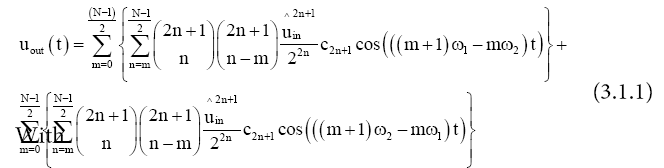- N as maximum Taylor series degree

- n as variable index

- m as degree of the generated intermodulation signals

- cn as taylor series coefficients

The share of the original signal (or main frequency) and IDMproducts various degrees (3 to 9) as follows: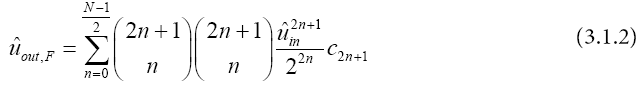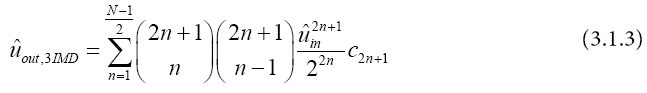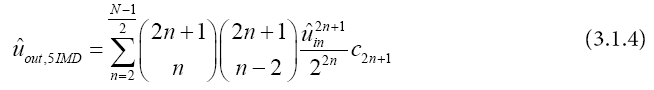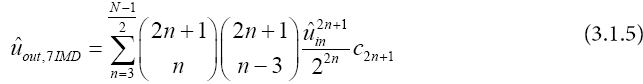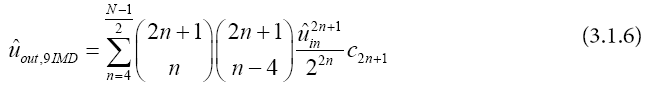To determine the coefficients series, linear equation system of considered signals using the measured values are written. The equation system for main signals (3.1.2), which is formed of the number M ,the corresponding measured values ûin and ûout , can be summarized as follows (Since the measured values on the left side (3.1.2) is real, the absolute terms of right side is used):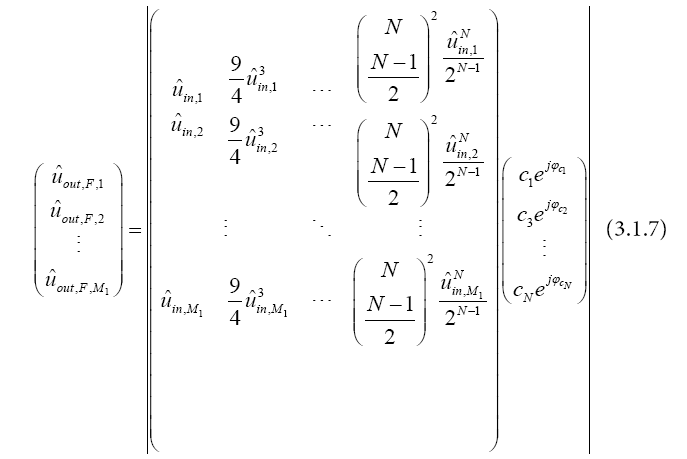The equation system for the 3IMD-products signals is too similar to the manner as the main signals equation and the basis of (3.1.3) for the M2 measured values can be summarized as follows: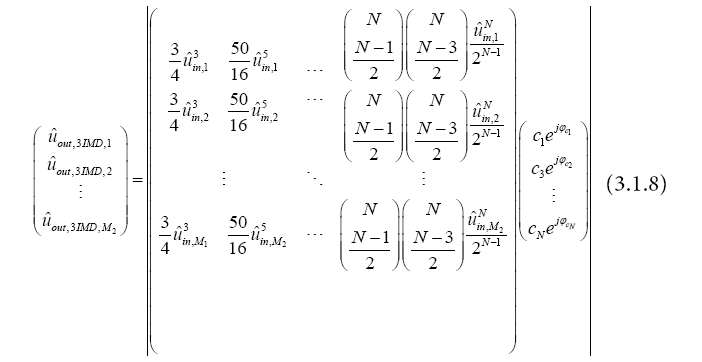The equation system of the intermodulation signals higher order are obtained same manner.

To write equations system related to the gain amplifier, the output voltage signal according to the input voltage with amplitude ûin and Frequency f11) is expended in Taylor series. In main frequency, output voltage can be summarized as follows: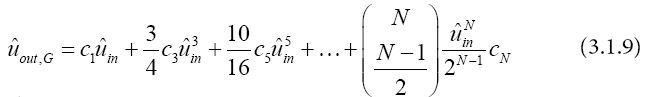The linear equation system of the gain amplifier for the M3 measured values as follows:

M2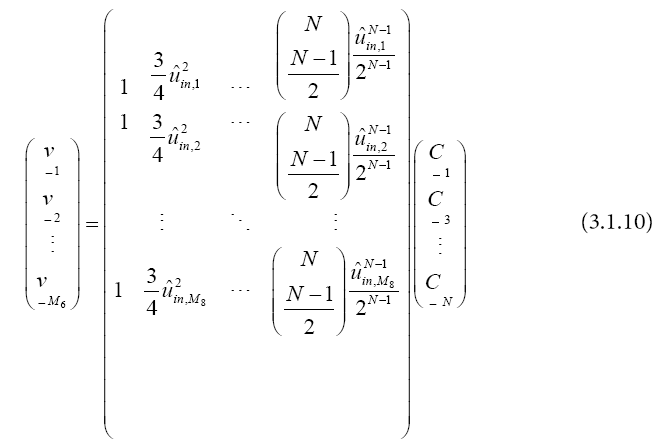Now magnitude and phase gain of amplifier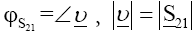should be regarded as real values separately. Since Taylor coefficients should satisfy all equations system, the individual linear equation system should be solved simultaneously, that is, the following linear equations system for M1, M2, M3, M4, M5, M6 measured values should be considered together: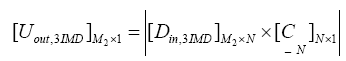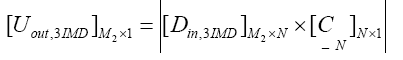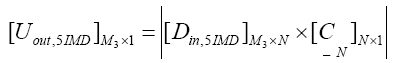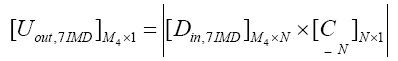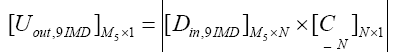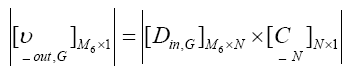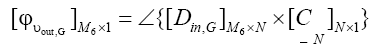With

Uout,F Uout,3IMD as output voltag for main signals, 3IDM-products, ... in (3.1.2), (3.1.3), ...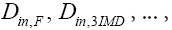as main matrix in (3.1.2), (3.1.3), ...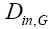as main matrix in (3.1.10) and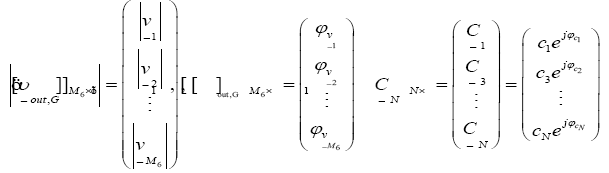The number of measurements M1, M2 etc. is not the same but has been selected depending on the available number of measurement points (length of the curve).

Optimization

To determine the complex coefficients, the transfer characteristics of the amplifier such as a model in the form of the mathematical approximation are presented; in other words using determine coefficients model, the difference between the model and measurements are minimized. To do this, the numerical optimization techniques are used. For optimization, the complex least square method is an appropriate technique, in which the model coefficients are determined through setting zero of the partial derivatives  that is: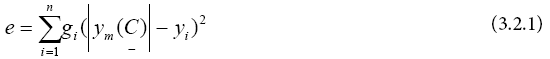And for phase relationship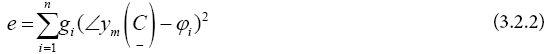With

e as error function,

yi as measured output value

ym as model (desired) output value

φi as measured output value for phase gain

gi as weighting function

n as the measured value and

C as model parameters that must be found

It is natural that all discussed equations system must be considered in error function. For this reason, cost function (CF) as a function of the total error and the sum of all the dividable functions (functions error) are included and formed. For 6 output signals, we have: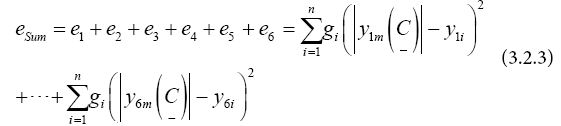Our research show that it is not possible to determine all the coefficients of the model with the same lowest error, especially the higher order ID-products can always be modeled worse . Because of that, the weighting function, (g1, g2….) is added in the cost function, the weighting factors for each output signal also be used so that be able to output signal or output signals with changes of this weights as requires to be optimized. So (3.2.3) is written in a new form as follows: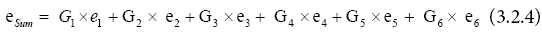The accuracy of the model depends on the series degree (n). A better accuracy can be achieved in principle by increasing of this value. However, it is difficult to enhance the performance for n > 13.

For the numerical solution of the optimization problem, the function “minsearch” to find the minimum of the cost function in MATLAB has been used. Examples of the measured results and of the model results can be seen in Figure 3(a), where the voltage gain magnitude and phase of amplifier A is plotted as a function of input power level for single-tone excitation, and in Figure 3(b), where the two-tone fundamental signal level and the intermodulation product levels are plotted versus input power level.

Figure 3 shows some deviations between model and measurements in the fundamental signal levels as well as in the intermodulation products; note that in the optimization of the model coefficients the largest weights were used for the fundamental and third-order intermodulation products.

Figure 3: Measured and modeled transfer characteristics of amplifier A: (a) Single-and two-tone gain, (b) two-tone fundamental and intermodulation pro ducts of orders 3, 5, and 7.

Figure 3(a) shows a good match for the magnitude and phase of the amplifier. Error obtained for the phase just a few degrees and for the magnitude is less than 0.5 dB. Figure 3(b) shows the divergence of the model when the two-tone input power goes beyond the highest input power level that was used as measurement data in the calculation of the Taylor series coefficients (-13.8 dB). Less dramatic but notable is the characteristic behavior below the divergence region: Deviations appear as slight oscillations with increasing amplitude closer to the divergence limit.

Using the mathematical model, it was possible to calculate the phase variation of the fundamental output signals for two-tone excitation, Figure 4, which was not accessible when measuring with a spectrum analyzer. Again, a slight oscillatory deviation is included in the calculated variation of the phase versus input power since a smooth parabolic-shaped variation should be expected. The observed oscillatory model errors, though not large on an average over the total extent of the modeled amplifier characteristics, will be found as major sources of error in the simulation of the new circuit, section 4.

Figure 4: Simulated phase variation of two-tone fundamental signals at amplifiers A and Bas a function of input power level.

#### Simulation of the New Concept

In order to understand the interdependencies in the intermodulation cancellation of the new circuit, the experimental circuit was simulated using the Taylor-series models for the two individual amplifiers and using scattering matrix models for the other components in the circuit (perfect match for all components assumed. Due to the high volume of the output signals of the amplifier A and B, discrete numerical methods (with help DFT - Discrete Fourier Transform) of the entire circuit in has been simulated in MATLAB which is more flexible than other software. Other simulation programs like ADS have been shown restrictions to model .

One obvious deviation of the real circuit from the simplified concept of section 2 is the considerable insertion loss (1.6 dB) of the two delay lines: With reference to the principle of operation and designations of signals and test points given in section II, in the first loop, this insertion loss attenuates the input signal before the sampled signal from amplifier A gets subtracted, which requires a reduction of the amplitude of the sampled signal (k ^ 2 < 2/υ) in order to achieve equal fundamental signal amplitudes at the input of both amplifiers. Also this reduces the amplitude of the sampled distortion signal s^' (t) from amplifier A that fed into amplifier B and the resulting distortion signal at the output of amplifier B will been reduced accordingly.

On the upper signal path, the upper delay line attenuates the fundamental and distortion signals in the same way before they appear at the combiner. After the above-described adjustment of the first loop coupling from amplifier A to amplifier B, the signals at the input of amplifier B can be compared to the input signals of amplifier A. Figure 5 presents the envelope peak voltages of the fundamental tones, of the third-order intermodulation products and of the total signal, normalized to the envelope peak voltage of the fundamental twotone signal at the input of amplifier A. It is seen that the fundamental tones have been adjusted to be equal in magnitude at both amplifiers for the “null” input power level of +3 dBm. At this input power level, the intermodulation products contribute already more than 10% of envelope voltage to the total input voltage of amplifier B, which presents a serious deviation from the assumption made in section II that the drive conditions of both amplifiers are basically equal.

Figure 5: Envelope peak voltage of the fundamental tones, the third-order intermodulation products and the total voltage at the input of amplifier B, all normalized to the input voltage of amplifier A.

Looking at lower drive levels, we find for the intermodulation products that the variation of voltage with drive power level exhibits an oscillatory error component as described in section 3; a more realistic indication of this variation is also given in the figure. The second notable effect is that the normalized fundamental signal voltage at amplifier B decays with reduced drive level. The reason becomes clear by inspecting Figure 3(a) and Figure 4: With reducing input power level the voltage gain of amplifier A increases and the insertion phase changes; thus, at the input of amplifier B, an increased signal sample from amplifier A is subtracted from the original input signal and creates a smaller fundamental signal component; the minimum around the drive level of -5 dBm is found to be due to the particular constellation of the amplification amplitude and phase variation with drive level.

Looking at the output side of both amplifiers, Figure 6 shows the amplitudes and phases of the fundamental and distortion signals at the power combiner: At the +3 dBm“null” drive level; we find the fundamental signal from amplifier B larger than that of amplifier A, which is due to the attenuation of upper delay line. At the same time, the two signals exhibit a considerable phase difference of 36° which in combination with the amplitude imbalance affects a combiner loss of about 0.5 dB; note that the power-added efficiency of the combiner circuit is further reduced by about 0.8 dB due to the dissipation loss of 1.6 dB from upper delay line affecting half the generated power of the power combiner.

Figure 6: Amplitude (a) and phase (b) of the fundamental two-tone signal and the third-order intermodulation product at the power combiner as a function of input power.

Instead of for best combining efficiency, the delay line phase and the first loop coupling in this simulation were optimized for intermodulation suppression, seen by approximately equal intermodulation product amplitudes at the “null” drive level and a close to 180° phase difference; note the oscillatory variation in the intermodulation magnitude plot of Figure 6(a), which again can be attributed to the approximation error in the single amplifiers’ Taylor series model. The intermodulation signal amplitudes are found approximately equal because the different effects of the insertion loss of the two delay lines in both loops nearly cancel, as both the intermodulation signals from amplifier A and of amplifier B get reduced in amplitude.

Again, turning to lower drive power levels, we observe about equal fundamental signal amplitudes at approx. -5 dBm input power, where Figure 5 has indicated a dip in the input signal of amplifier B, thus compensating the amplitude imbalance due to the upper delay line attenuation. At even lower drive level, the input signal amplitude of amplifier B recovers from the dip and the fundamental signal from amplifier B becomes larger again than that of amplifier A. At the same time, due to the reduced fundamental signal drive of amplifier B relative to amplifier A, this amplifier produces considerably less intermodulation (s"(t)) than amplifier A, approximately a 3 dB reduction for 1 dB reduction in drive power. The combination of the two intermodulation components -2s′(t)+s″(t) at the output of amplifier B thus increases in magnitude as the drive power is reduced and the gain of amplifier A increases. With growing difference in the two intermodulation contributions at the combiner, the circuit loses its cancellation effect.

#### Measurement of the Proposed Amplifier Circuit

Before building an experimental new amplifier circuit, two power amplifiers were assembled and tested: The amplifiers used 900 MHz silicon FET power modules MHW916 in cascade with preamplifiers which gave a saturated output power of about 14 W at a gain of about 53 dB. Measurement of the forward transmission group delay was performed with single-tone at small-signal level using a vector network analyzer HP8722C and the result was used to specify the length of the two delay lines in the new amplifier circuit. Measurements of the complex valued single-tone voltage gain (scattering coefficient S21) as a function of input power level were performed using the vector network analyzer and two tone measurements (910 MHz and 911 MHz) of the fundamental signals and up to the seventh-order intermodulation product were performed using a spectrum analyzer HP8565E (Figures 7 and 8). Both sets of measurements were used for the modeling of the amplifiers transfer characteristics based on a Taylor-series expansion with complex coefficients.

Figure 7: The experimental single-tone voltage gain, (a) sketch of setup and (b) photograph of bench equipment and amplifier circuit.

Figure 8: The measured fundamental signals and up to the seventh-order intermodulation product, (a) sketch of setup and (b) photograph of bench equipment and amplifier circuit.

The measured fundamental signals and up to the seventh-order intermodulation product, (a) sketch of setup and (b) photograph of bench equipment and amplifier circuit (Figure 8).

The setup Sketch of the experimental proposed amplifier circuit (called the feed forward power combiner circuit) is presented in Figure 9. The fundamental two-tone input signal is produced by combining two signal generators and the input signal split of 1:3 voltage ratio is realized by a -3 dB divider with a 10 dB attenuator in the upper signal path to amplifier A. All components of the power combiner circuit are connected by coaxial cables which introduce some insertion loss and phase shift. In particular, the required time delays are realized by about 4 m long coaxial cables RG213 which introduce about 1.6 dB of extra loss. Between the two directional couplers a variable attenuator and a variable phase shifter are inserted in order to allow compensation of amplitude and phase offsets in the first loop. At the output side, a power attenuator is inserted between the power combiner and the spectrum analyzer in order to save the instrument from damage by high incident power.

Figure 9: (a)The measured proposed amplifier circuit and (b) the sketch of setup and (c) photograph of bench equipment and amplifier circuit.

Adjustment of the circuit turned out to be difficult: In particular, the variation of the voltage gain in magnitude and phase as a function of input power level, as seen in Figures 3 and 4, required selecting the drive power level for optimum linearity first of all. In our proof of concept experiment, we set the input power level to +3 dBm which corresponds to the 1 dB-saturation level of the individual power amplifiers.

With the drive signal level fixed, the signal at point B1 at the input of amplifier B was observed using a spectrum analyzer and the upper delay line was adjusted and the variable attenuator and phase shifter were set such that the fundamental two-tone signals were approximately equal in level to the signals at point A1 at the input to power amplifier A. This setting, at the same time gives approximately the correct pre-distortion level necessary for intermodulation cancellation at the second loop. Final adjustment of the variable attenuator and phase shifter was based on the measurement of the signals at the combiner output; either an optimum cancellation of the third-order intermodulation products could be achieved with the fundamental signals from amplifiers A and B slightly unequal in amplitude and phase or nearly equal fundamental signals could be achieved with considerable difference in phase and amplitude of the intermodulation products. When the amplifier circuit was adjusted for optimum intermodulation cancellation, phase- and amplitude deviations gave rise to a loss in fundamental signal output power of about 0.4 dB.

In Figure 10, the measurement of the proposed amplifier characteristic is presented and is compared to the expected characteristic of the conventional power combiner circuit using two amplifiers type “A” in parallel if excited at the same input power level as the amplifiers in the proposed amplifier circuit.

Figure 10: Measured transfer characteristic of the experimental new amplifier circuit (feed forward-power combiner circuit) compared to the expected characteristic of a conventional parallel power combining amplifier.

It is seen that the proposed amplifier achieves a notable extension of the linear range for the fundamental signals around the 1 dB compression level of the individual power amplifiers while the thirdorder intermodulation products are reduced by 25 and 45 dB at the +3 dBm input level (the two 3IMD-products are unequal, as explained in reference ). However, the cancellation is confined to a limited range of power levels around the “null”-input level and the intermodulation products level is not improved relative to the level of the conventional parallel power combiner outside this narrow region and is even worse in some parts of the input level range. This is a fundamental limitation of the feed forward power combiner compared to the conventional feed forward amplifier which was investigated by simulation in section 4. For a practical application of the feed forward power combiner concept, this means that in an operation mode with dynamically changing drive conditions, e.g., changing numbers and levels of modulated carriers as in a mobile communications base station amplifier, the loop adjustment has to be adapted dynamically also. Otherwise, under more static drive conditions, as, e.g., in TVsatellite power amplifiers, the feed forward cancellation concept could improve the linearity of present parallel power combining amplifiers with only moderate adaptivity requirements.

#### Conclusion

The limitation of the feed forward power combiner circuit was shown to be due to the saturation effect of the upper power amplifier, with its gain variation offsetting the balancing of the circuit loops. However, driving the power amplifiers into the saturation range is a necessary condition for high power efficiency. Power-added efficiency of our experimental amplifier was about 36% which is in contrast to around 10% efficiency of conventional FF-amplifiers. As a price, the critical drive level dependence of the combiner circuit may require higher adaptivity and control of the loop adjustments than a conventional feed forward amplifier, depending on the dynamics of the signals to amplify. By simulation, it can be shown that at lower drive powers the limitations get weaker as the intermodulation cancellation exhibits broader “null” and cancellation is improved even far outside the “null”, similar to the characteristics of the conventional feed forward amplifier, yet losing the advantage in power efficiency.

#### References

Select your language of interest to view the total content in your interested language

### Article Usage

• Total views: 2133
• [From(publication date):
February-2017 - Aug 26, 2019]
• Breakdown by view type
• HTML page views : 1972
• PDF downloads : 161

## Post your commentCan't read the image? click here to refresh
###### Peer Reviewed Journals

Make the best use of Scientific Research and information from our 700 + peer reviewed, Open Access Journals

International Conferences 2019-20

Meet Inspiring Speakers and Experts at our 3000+ Global Annual Meetings

Top